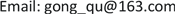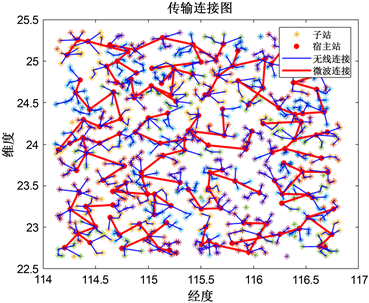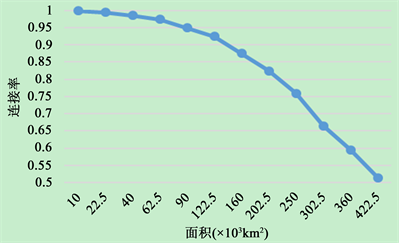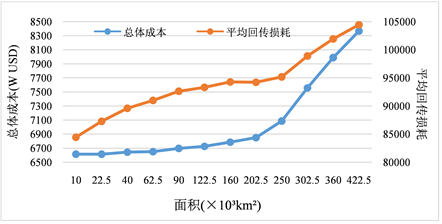﻿ 双目标无线回传拓扑整数规划模型及算法 Integer Programming Model and Algorithms for Dual-Objective Wireless Return Topology

Computer Science and Application
Vol. 09  No. 12 ( 2019 ), Article ID: 33291 , 7 pages
10.12677/CSA.2019.912250

Shaohu Wang, Yuhui Hu, Xuanhao Yang, Qu Gong

College of Automation, Chongqing University, ChongqingReceived: Nov. 14th, 2019; accepted: Nov. 29th, 2019; published: Dec. 5th, 2019ABSTRACT

In the actual Relay deployment, the layout and connection of the sites are limited by various factors such as cost, distance, and backhaul quality, which bring challenges to the topology planning of the sites. Aiming at the problem of layout planning of communication base stations, a dual-objective station integer programming model is established, and a block partitioning algorithm based on K-means clustering algorithm and a site connection algorithm based on Prim algorithm are designed. The results of simulations show that the model and algorithms can effectively solve the problem of site planning in wireless backhaul topology, and it has better applicability and effectiveness while reducing the complexity of the algorithms.

Keywords:Wireless Backhaul Topology, Integer Programming, K-Means Clustering Algorithm, NP-Hard Problem1. 引言

2. 系统模型

xi表示站点类型， ${x}_{i}=0$ 表示i站点为子站， ${x}_{i}=1$ 表示该站点为宿主站； ${y}_{ij}$ 表示站点间的连接关系， ${y}_{ij}=0$ 表示i与j站点没有连接关系， ${y}_{ij}=1$ 表示站点间存在连接关系；e为宿主站的类型， $e=1$ 表示站型为RuralStar， $e=2$ 表示站型为蝴蝶站，RuralStar包含1个扇区，蝴蝶站包含2个扇区。 ${S}_{ij}$ 表示i与j站点间的距离：

${S}_{ij}=R\cdot \mathrm{arccos}\left[\mathrm{cos}{\beta }_{i}\mathrm{cos}{\beta }_{j}\mathrm{cos}\left({\alpha }_{i}-{a}_{j}\right)+\mathrm{sin}{\beta }_{i}\mathrm{sin}{\beta }_{j}\right]$ (1)

2.1. 规划目标

$\mathrm{min}P=\underset{i=1}{\overset{N}{\sum }}{x}_{i}{P}_{1}+\underset{i=1}{\overset{N}{\sum }}\left(1-{x}_{i}\right){P}_{2}+W{P}_{3}$ (2)

$P{L}_{ij}=\left(1-{x}_{i}{x}_{j}\right)\left[32.5+20\mathrm{log}\left({S}_{ij}\right)+20\mathrm{log}\left(F\right)\right]$ (3)

$\mathrm{min}\underset{i=1}{\overset{N}{\sum }}\underset{j=1}{\overset{N}{\sum }}P{L}_{ij}{y}_{ij}$(4)

2.2. 站点连接中的约束条件

1) 回传距离约束

${x}_{i}\left(1-{x}_{j}\right){y}_{ij}{S}_{ij}\le Di{s}_{1}$ (5)

$\left(1-{x}_{i}\right)\left(1-{x}_{j}\right){y}_{ij}{S}_{ij}\le Di{s}_{2}$ (6)

${x}_{i}{x}_{j}{y}_{ij}{S}_{ij}\le Di{s}_{3}$ (7)

2) 站点间连接的拓扑约束 

1˚站点包含RuralStar和蝴蝶站两种不同站型；其中，RuralStar共包含1个扇区，蝴蝶站共包含2个扇区  ；若该站点为宿主站，则每个扇区第一级子站数目和子站总个数存在限制。表示如下：

$\underset{j=1}{\overset{N}{\sum }}{x}_{i}\left(1-{x}_{j}\right){y}_{ij}\le Nu{m}^{\left(1\right)}e$ (8)

$\underset{i\in {C}_{k}}{\sum }\left(1-{x}_{i}\right)\le Nu{m}^{\left(2\right)}e$ (9)

2˚每个子站最多只能有2条无线回传连接：

$\underset{j=1}{\overset{N}{\sum }}\left(1-{x}_{i}\right){y}_{ij}\le 2$(10)

3) 宿主站间成片连接约束

$Ceil\left(\frac{{\sum }_{{a}_{i}\in {L}_{t}}{x}_{i}}{Nu{m}^{\left(3\right)}}\right)\le {W}_{t}$ (11)

$\left\{\begin{array}{l}\mathrm{min}P=\underset{i=1}{\overset{N}{\sum }}{x}_{i}{P}_{1}+\underset{i=1}{\overset{N}{\sum }}\left(1-{x}_{i}\right){P}_{2}+W{P}_{3}\\ \mathrm{min}\underset{i=1}{\overset{N}{\sum }}\underset{j=1}{\overset{N}{\sum }}P{L}_{ij}{y}_{ij}\end{array}$

$\text{s}\text{.t}\text{.}\left\{\begin{array}{l}\left(1\right),\left(2\right),\left(3\right),\left(4\right),\left(5\right),\left(6\right),\left(7\right),\left(8\right),\left(9\right),\left(10\right),\left(11\right)\\ {x}_{i},{y}_{ij}\in \left\{0,1\right\}\end{array}$

3. 求解算法

$K=ceil\left(\frac{N}{m}\right)$ (12)

${m}_{j}=\frac{1}{|{C}_{j}|}\underset{i\in {C}_{j}}{\sum }{\alpha }_{i},\text{\hspace{0.17em}}\text{\hspace{0.17em}}{n}_{j}=\frac{1}{|{C}_{j}|}\underset{i\in {C}_{j}}{\sum }{\beta }_{i}$ (13)

Step 1 利用K-means聚类算法将N个站点划分为M个区块： ${D}_{1},{D}_{2},\cdots ,{D}_{M}$

Step 2 对每个区块求出最短的可行连接方案。

Step 2.1 令i = 1；

Step 2.2 在Di中任取一个站点作为宿主站，记为v0。令 $U←\left\{{v}_{0}\right\}$$V←{S}_{i}-U$，j = 0；

Step 2.3 若 $j>Nu{m}^{\left(1\right)}e$，则转Step 2.6，否则从V中找到与U中v0所关联的距离最小的边，若该边小于 $Di{s}_{1}$，则将该边加入Ei，将其关联的站点v1加入到U中， $j←j+\text{1}$，令 $V←V-\left\{{v}_{1}\right\}$，转Step 2.4；否则转Step 2.6。

Step 2.4 从V找与U中v1所关联的距离最小的边，若该边小于 $Di{s}_{2}$，则将该边加入Ei，将关联的站

Step 2.5 从V开始找与U中v2所关联的距离最小的边，若该边小于 ，则将该边加入Ei，将关联的站点v3加入到U中，并且从V中删除，否则返回Step 2.3；

Step 2.6在区块中另设一个宿主站，记为v0，重复Step 2.2至Step 2.5，直到区块中所有站点遍历为宿主站，得到区块 ${D}_{k}$ 所有可行连接方案，记为 ${P}_{k}=\left\{{P}_{k}^{1},{P}_{k}^{2},\cdots ,{P}_{k}^{|{D}_{k}|}\right\}$。其中， ${P}_{k}^{i}$ 表示区块 ${D}_{k}$ 内以i站点作为宿主站得到的连接方案。 $i←i+\text{1}$，若i < M，返回Step 2.1，否则，下一步。

Step 3 宿主站确定。

Step 3.1 K-means聚类分析确定二级区块：将一级区块中心点聚类为 $Nu{m}^{\left(3\right)}$ 个区块中心点的二级区块 ${L}_{t}$，以保证尽可能多的宿主站共用同一颗卫星；

Step 3.2 利用Prim算法确定二级区块中宿主站间的成片连接关系，之后判断每条边的长度是否小于 $Di{s}_{3}$，若成立，则保留，否则将该条边打断。得到二级区块 ${L}_{t}$ 中任意一级区块宿主站可行方案组合下的卫星数量：

$Nu{m}^{{L}_{t}}\left({P}_{k}^{{i}_{k}},{P}_{k+1}^{{i}_{k+1}},\cdots ,{P}_{k+Nu{m}^{\left(3\right)}-1}^{{i}_{k+Nu{m}^{\left(3\right)}-1}}\right)$

$P{L}^{{L}_{t}}\left({P}_{k}^{{i}_{k}},{P}_{k+1}^{{i}_{k+1}},\cdots ,{P}_{k+Nu{m}^{\left(3\right)}-1}^{{i}_{k+Nu{m}^{\left(3\right)}-1}}\right)$

Step 3.3双目标规划模型求解确定宿主站。基于Step 3.1和Step 3.2的结果，建立宿主站双目标规划模型确定宿主站的位置，针对每一个二级区块，卫星数量越少则总费用越小，同时需要使得回传损耗尽可能最小。模型如下：

$\left\{\begin{array}{l}\mathrm{min}Nu{m}^{{L}_{t}}\left({P}_{k}^{{i}_{k}},{P}_{k+1}^{{i}_{k+1}},\cdots ,{P}_{k+Nu{m}^{\left(3\right)}-1}^{{i}_{k+Nu{m}^{\left(3\right)}-1}}\right)\\ \mathrm{min}P{L}^{{L}_{t}}\left({P}_{k}^{{i}_{k}},{P}_{k+1}^{{i}_{k+1}},\cdots ,{P}_{k+Nu{m}^{\left(3\right)}-1}^{{i}_{k+Nu{m}^{\left(3\right)}-1}}\right)\end{array}$

s.t.

$\underset{{i}_{k}\in {C}_{k}}{\sum }{x}_{{i}_{k}}=1$

4. 仿真结果及分析Figure 1. Final station plan diagram

1000个候选站点的规划方案为：宿主站共143个，卫星共18个，连接关系如图1所示。输出结果中，94.5%的站点都纳入规划，说明了算法具有很高的可靠性。算法输出结果的总成本为6660 WUSD，总的回传损耗为88,220。Figure 2. Site distribution area and site connection rate curveFigure 3. Site distribution area and overall cost/return loss curve

5. 结论

Integer Programming Model and Algorithms for Dual-Objective Wireless Return Topology[J]. 计算机科学与应用, 2019, 09(12): 2249-2255. https://doi.org/10.12677/CSA.2019.912250

1. 1. 詹子群. 关于通信运营商无线基站建设设计方面的探讨[J]. 电子测试, 2019(6): 64-65.

2. 2. 董江波, 程伟, 陈燕雷, 韩云波. Relay技术引入对无线网络规划的思考与研究[J]. 电信工程技术与标准化, 2013, 26(7): 23-28.

3. 3. 石朗昱, 马玲. Relay无线回传技术在TD-LTE组网方案中的应用研究[J]. 电信工程技术与标准化, 2017, 30(2): 41-43.

4. 4. 张青松. 基站站址规划在通信基础设施城乡规划中的应用[J]. 通信电源技术, 2018, 35(10): 210-213.

5. 5. 石雷, 刘正丽, 韩江洪, 石怡, 魏振春. 基于干扰管理的高吞吐无线多跳网络基站选址算法[J]. 电子测量与仪器学报, 2016, 30(3): 389-399.

6. 6. 张宏远, 谷寒雨, 席裕庚. 基于聚类分解的WCDMA基站布局规划算法[J]. 控制与决策, 2006, 21(2): 213-216.

7. 7. 南雪莹. 基于粒子群算法的Relay无线回传方案部站优化[J]. 中国新通信, 2019, 21(3): 128.

8. 8. 张然. 移动回传网络的可靠性部署研究[D]: [硕士学位论文]. 北京: 北京邮电大学, 2016.

9. 9. Dinwoodie, J. (1996) Network and Discrete Location: Models, Algorithms and Applications. Journal of Transport Geography, 4, 302-303. https://doi.org/10.1016/S0966-6923(97)89394-5

10. 10. Lee, S., Lee, S.K., Kim, K. and Kim, Y.H. (2015) Base Station Placement Algorithm for Large-Scale LTE Heterogeneous Networks. PLoS ONE, 10, e0143265. https://doi.org/10.1371/journal.pone.0139190

11. 11. Monti, P., Tombaz, S., Wosinska, L. and Zander, J. (2012) Mobile Backhaul in Heterogeneous Network Deployments: Technology Options and Power Con-sumption. 2012 14th International Conference on Transparent Optical Networks, Coventry, UK, 2-5 July 2012, 1-7. https://doi.org/10.1109/ICTON.2012.6253839

12. 12. 马梁龑. 无线Relay传输技术在微基站部署中的策略[J]. 移动通信, 2017, 41(24): 13-18.

13. 13. 章永来, 周耀鉴. 聚类算法综述[J/OL]. 计算机应用: 1-14.

14. 14. 李建军, 沈啸林, 陈明贺, 刘硕, 陈舒研. 基于最小生成树算法的怀柔区快递站点选址问题研究[J]. 南方农机, 2019, 50(1): 91-93.

15. 15. 曾宪昌. 求解智能问题的模拟仿真法[J]. 数学物理学报, 1983(4): 385-394.